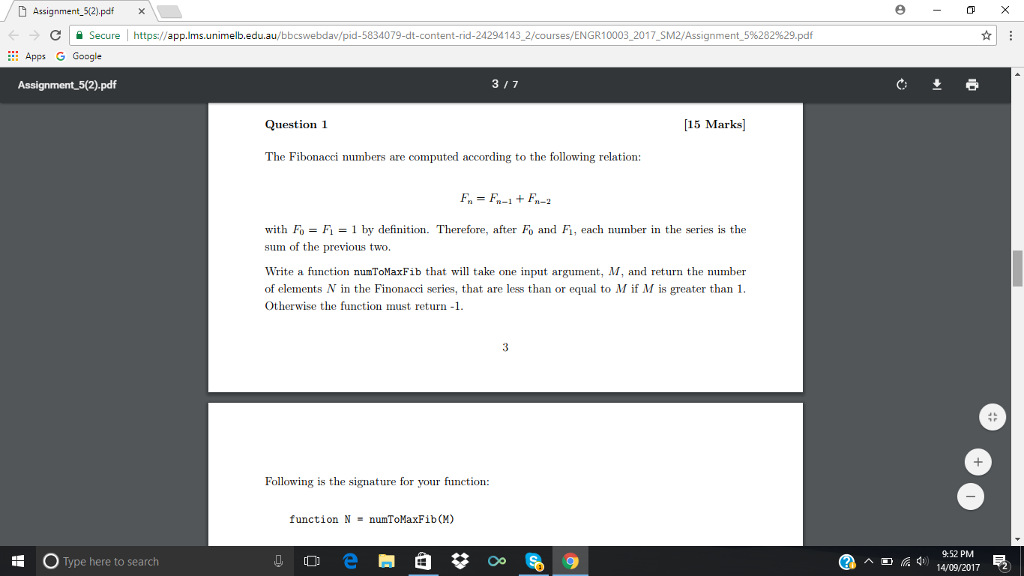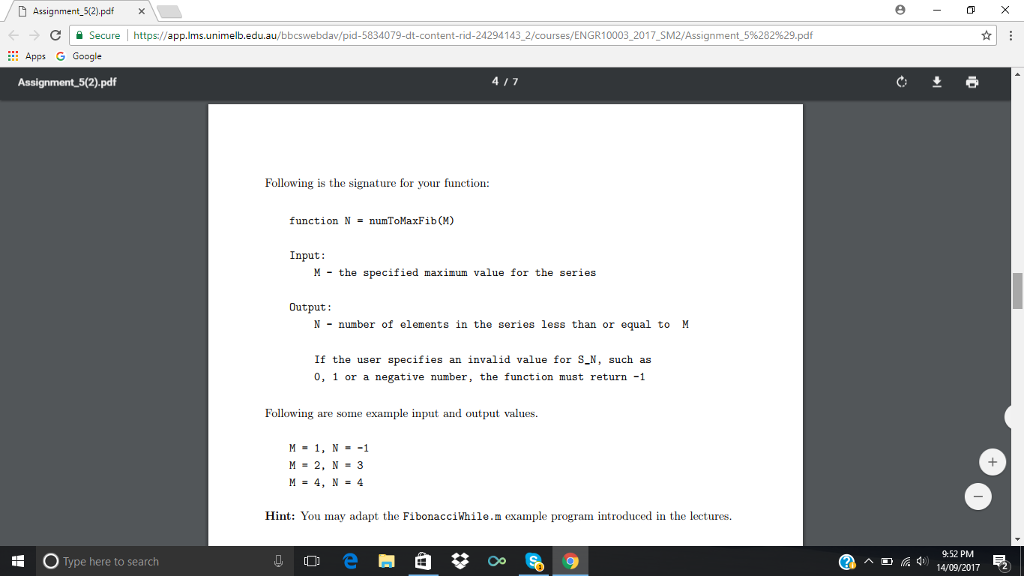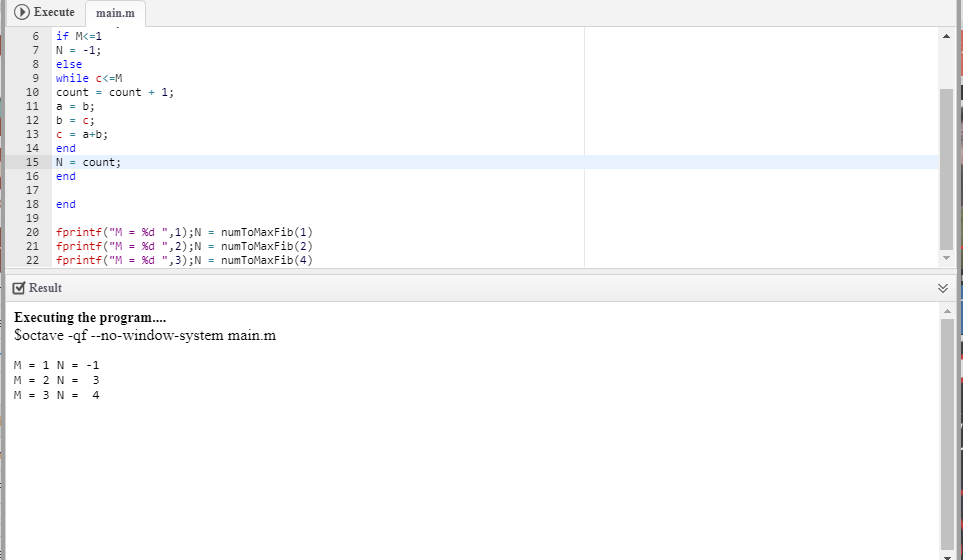# Question & Answer: DAssignment5(2).pdf Secure https://app.lms.unimelb.edu.au/bbcswebdav pid-5834079-di tent-rid-24294 1 43-2/courses/E…..Don't use plagiarized sources. Get Your Custom Essay on
Question & Answer: DAssignment5(2).pdf Secure https://app.lms.unimelb.edu.au/bbcswebdav pid-5834079-di tent-rid-24294 1 43-2/courses/E…..
GET AN ESSAY WRITTEN FOR YOU FROM AS LOW AS \$13/PAGE

DAssignment5(2).pdf Secure https://app.lms.unimelb.edu.au/bbcswebdav pid-5834079-di tent-rid-24294 1 43-2/courses/ENGR1 0003-2017-SM2/Assignment-5%2 Apps G Google 3 17 Question [15 Marks The Fibonacci numbers are computed according to the following relation with Fo- Fi-1 by definition. Therefore, after Fo and Fi, each number in the series is the sum of the previous two. Write a function nunToMaxFib that wll take one input argument, M, and return the number of elements N in the Finonacci series, that are less than or equal to M if M is greater than 1 Otherwise the function must return1 Following is the signature for your function: function N = numToMaxF1b (M) 952 PM Type here to search dy) 14/09/2017 屍 ^

function N = numToMaxFib(M)
a = 0;
b = 1;
c = a+b;
count = 1;
if M<=1
N = -1;
else
while c<=M
count = count + 1;
a = b;
b = c;
c = a+b;
end
N = count;
end

end

fprintf(“M = %d “,1);N = numToMaxFib(1)
fprintf(“M = %d “,2);N = numToMaxFib(2)
fprintf(“M = %d “,3);N = numToMaxFib(4)

Output:-